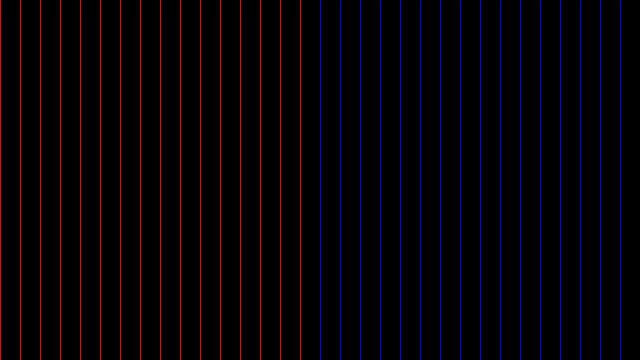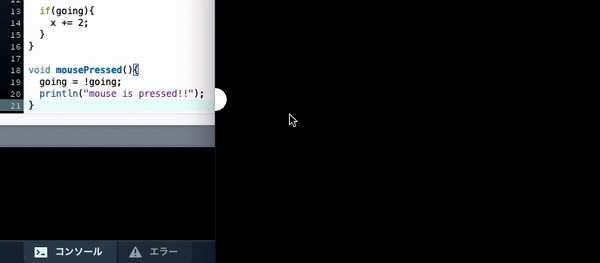# [Java] [Processing x Java] Data types and object-oriented programming

1. Data type
2. Object-oriented programming

# 0.Data type

## 0-0. What is the data…?

Before we talk about data types, let’s talk about “data”. Data is information. Weight, height, eye color, car number, sunshine hours, precipitation, address, images, etc. .. In program terms, variables are data, functions are data, and classes are data.

Point: Data is represented in computer memory as a set of bits (0 and 1). Point: Data has a type (** data type **) and is stored by type.

Data types (data types) include Basic data type and Complex data type.

###[Basic data type] The basic data type stores “Only one data element”.

Name Data Element Size (bits) Value Range
boolean boolean 1 true or false
byte byte 8 -128 to 127
char 1 alphanumeric character, symbol 16 0 to 65535
int integer 32 -2,147,483,648 to 2,147,483,647
float Floating point numbers 32 3.40282347E + 38 to -3.40282347E + 38
color color 32 16,777,216 colors

###[Composite data type] A complex data type contains “multiple data or functions (methods)”. *Composite data types are also called classes.

Name Data Element Method
String Character string length(),charAt(),equals(),indexOf(),toLowerCase(),toUpperCase()…
PFont Font list()
PShape Shapes beginShape(),endShape(),translate(),rotate(),scale()…
:

*Class names start with a capital letter, so they can be easily distinguished from basic data types.

## 0-2. Data type usage example

[Basic data type]

### Data type: boolean

####◯ A program to change the color of the line by using boolean

#### `boolean01.java`

``````
// Variable declaration and initialization
boolean b = false;

size(640, 360);
background(0);
//Build-in variable width can be used only after it is defined by size().
int middle = width/2;

// Loop to draw a line
for (int i = 0; i <= width; i += 20) {
//Determine when it is true and when it is false.
if (i <middle) {
b = true;
} else {
b = false;
}
// if true
if (b == true) {
stroke(255,0,0);
}
// if false
if (b == false) {
stroke(0,0,255);
}
// draw a line
line(i,0,i,height);
}
``````####◯ A program that controls the movement of the circle by pressing the mouse

#### `boolean02.java`

``````
//Define a float type variable x that indicates the x coordinate of the circle.
float x = 0;
//Define a boolean variable going that determines whether or not the circle advances.
boolean going = false;

void setup(){
size(500,500);
}
// infinite loop (create animation)
void draw(){
background(0);
fill(255);
// Coordinates of circle center = (x,height/2)
ellipse(x,height/2,30,30);

// if going = True
if(going){
x += 2;
}
}

// executed once when the mouse is pressed
void mousePressed(){
//To going, assign the boolean value opposite to the current boolean value.
// If going is True, assign False.
//If going is False, assign True.
going = !going;
// Output to the console when the mouse is pressed.
println("mouse is pressed!!");
}
``````### Data type: char

####◯ A program that efficiently outputs characters using a loop

#### `char.java`

``````
//Create a char type variable letter and initialize it.
char letter ='A';

//Char type has character (alphabet) order, so use it.
for(int i = 0;i <26;i++){
print(letter);
// Add characters one by one
letter++;
}
``````
``````ABCDEFGHIJKLMNOPQRSTUVWXYZ
``````

### Data type: int

####◯ Program that efficiently outputs multiples using a loop

#### `int.java`

``````
// Declare int type variable n and initialize.
int n = 3;

//5 repeat loop
for(int i = 0;i <5;i++){
println(n);
// n = 2n
n *= 2;
}
``````
``````3
6
12
twenty four
48
``````

### Data type: color

#### `color.java`

``````
//Define the color type variable blue using color().
color blue = color(0,0,255);
background(blue);
``````[Composite data type]——————————————— ——————–

### Data type: String

####◯ Program that uses the method of String class

#### `String.java`

``````
//Create an object s1 of class String and insert the contents.
String s1 = "breakfast"
// Use dot (.) to access the method of the object.
println(s1.length());
``````
``````9
``````

s1 is a String class object, and it is an instance variable in terms of variables.

### Data type: PImage

####◯ Program that displays images

#### `PImage.java`

``````
// object declaration
//Embodi the PImage class and create an object called img.
PImage img;

void setup(){
size(100,100);
// Put the contents in the object.
}

void draw(){
// use an object
image(img,0,0);
}
``````

# 1. Object oriented programming

## 1-0. First, check the terms

Object oriented programming A program that collectively defines related functions and variables.

class A collection of related fields and methods. It is often compared to a recipe.

field A variable defined in the class.

Method A function defined in a class.

object It is an instance (a concrete example) of a class. It has the same field names and methods as the original class. It can be likened to dishes that are often made using recipes.

instance An object of a certain class.

Instance variable A type of variable. The variable created when the object was created.

variable An element of data with a name. Every variable has a value, a data type, and a scope.

scope The range (block) in a program where variables can be accessed. The place where it can be used depends on where it is defined.

## 1-1. ** Programming with classes **

###◯ I want to use the class

Since the class is just a template, it cannot be used as it is. So

** “Increment a class to create an object and treat it like a variable while taking advantage of the characteristics of the object” **

I will do so. ###◯ Concrete class

The class describes the fields and methods. I want to use this in a program. But the class is just a template, an abstract entity.So, let’s make a class a concrete entity (object) and operate on it.

###◯ How to embody a class Let’s take a look at a simple example. Here, object s1 is created from class String.

#### `String.java`

``````
// object declaration
String s1;
// object definition
s1 = "breakfast"
// use the object
println(s1.length());
``````
``````9
``````

Where s1 is Object (a concrete entity created from a class) And Instance variable (variable created when the instance is created) is.

The name (appearance) differs depending on where you look from and where you set the standard, but the contents are the same. **There are various names. **

###◯ Treat it like a variable while taking advantage of the characteristics of the object

All objects have the fields and methods that the class has.

Field = a variable defined in the class

Method = function defined in the class

Now that we have an object, we can finally use the fields and methods of the class.

Use the dot (.) to access the fields and methods of the object. The object itself is treated like a variable.

#### `String.java`

``````
// object definition
String str1 = "goodjob";
// access the methods that the object has
str1 = str1.toUpperCase();
// use the object
println(str1);
``````
``````GOODJOB
``````

To use like a variable **Definition (Declaration → Initial Setting) → Use ** Is to be used like.

So in summary, str1 here is

□ Has fields and methods. (Characteristics of objects) □ Use dots to access your fields and methods. (How to use) And □ Has a value, data type, and scope. (Characteristics of variables) □ Use after defining (declaration, initial setting). (How to use)

These are just some of the ways you can program with classes.